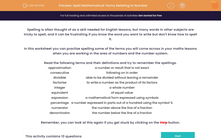# Spell Mathematical Terms Relating to Number

In this worksheet, students will practise spelling terms relating to numbers and the number system.Key stage:  KS 3

Curriculum topic:   Grammar and Vocabulary

Curriculum subtopic:   Vocabulary and Grammar Awareness

Difficulty level:#### Worksheet Overview

Spelling is often thought of as a skill needed for English lessons, but many words in other subjects are tricky to spell, and it can be frustrating if you know the word you want to write but don't know how to spell it.

In this activity, we will practise spelling some of the terms you will come across in your maths lessons when you are working in the area of numbers and the number system.Read the following terms and their definitions and try to remember the spellings.

 Approximation A number or result that is not exact Consecutive Following on in order Divisible Able to be divided without leaving a remainder Factorise To write a number as the product of its factors Integer A whole number Equivalent Of equal value Expression A mathematical form expressed using symbols Percentage A number expressed in parts out of a hundred using the symbol % Numerator The number above the line of a fraction Denominator The number below the line of a fraction

Remember, you can look at this again if you get stuck by clicking on the red help button on the screen.